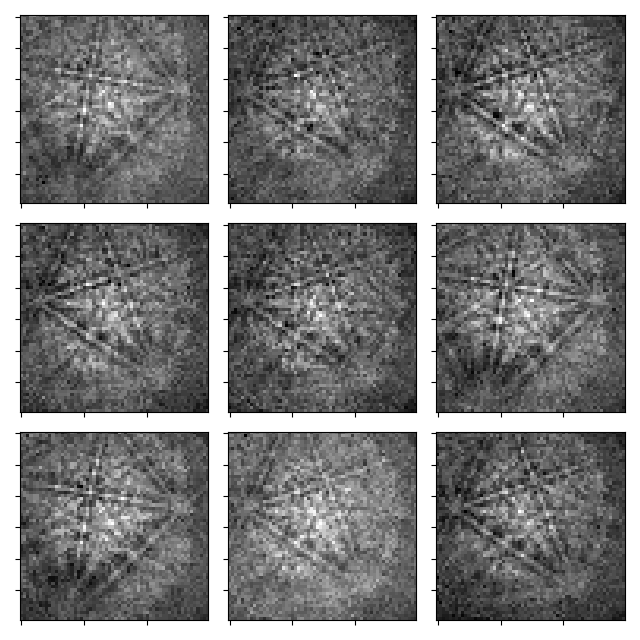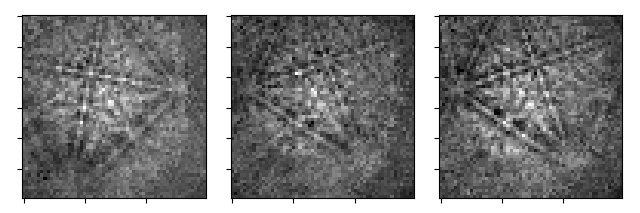This example shows various ways to crop the navigation axes of an `EBSD` signal using HyperSpy’s `inav` slicer and `crop()` method (see Indexing for details).

```import hyperspy.api as hs
import kikuchipy as kp

# Import data
s = kp.data.nickel_ebsd_small()
s.remove_static_background(show_progressbar=False)

# Inspect data and attributes
plot_kwds = dict(axes_decor=None, label=None, colorbar=None, tight_layout=True)
_ = hs.plot.plot_images(s, **plot_kwds)
print(s)
print(s.xmap.shape)
``````<EBSD, title: patterns Scan 1, dimensions: (3, 3|60, 60)>
(3, 3)
(3, 3)
```

Get a new signal with the patterns in the first row using `inav`. Note how the `xmap` and `detector` attributes are updated.

```s2 = s.inav[:, 0]

_ = hs.plot.plot_images(s2, **plot_kwds)
print(s2)
print(s2.xmap.shape)
``````<EBSD, title: patterns Scan 1, dimensions: (3|60, 60)>
(3,)
(3,)
```

Get the first column using `crop()`, which overwrites the signal inplace

```s3 = s.deepcopy()
s3.crop(1, start=0, end=1)

_ = hs.plot.plot_images(s3, **plot_kwds)
print(s3)
print(s3.xmap.shape)
``````<EBSD, title: patterns Scan 1, dimensions: (3, 1|60, 60)>
(1, 3)
(1, 3)
```

While `inav` returned a signal with only one navigation dimension, `crop()` left a single row. We can remove this `(1,)` dimension using `squeeze()`, but note that the custom `EBSD` attributes are not cropped accordingly

```s4 = s3.squeeze()

print(s4)
print(s4.xmap.shape)
```<EBSD, title: patterns Scan 1, dimensions: (3|60, 60)>